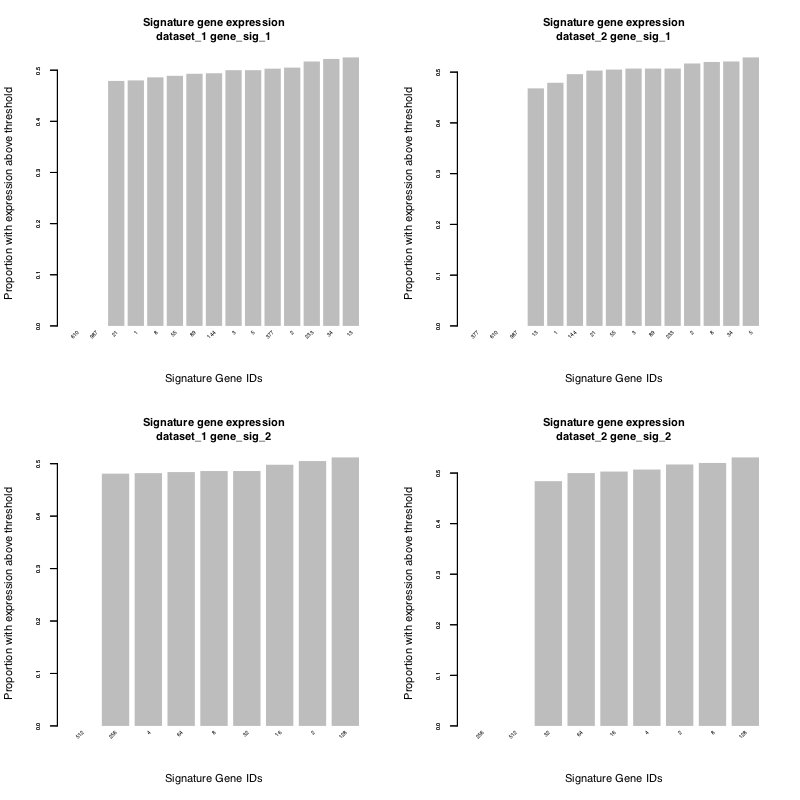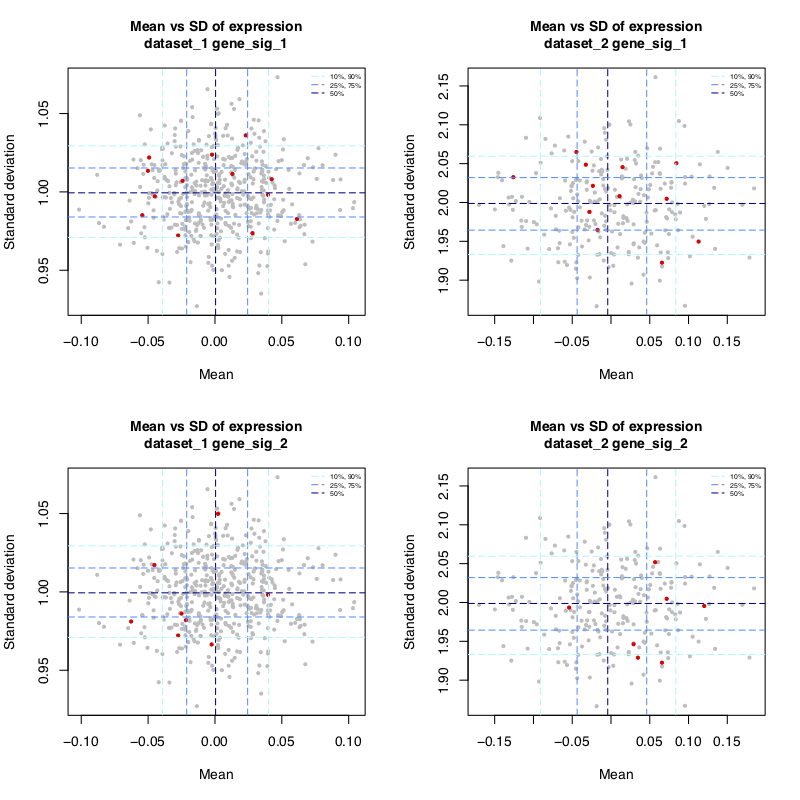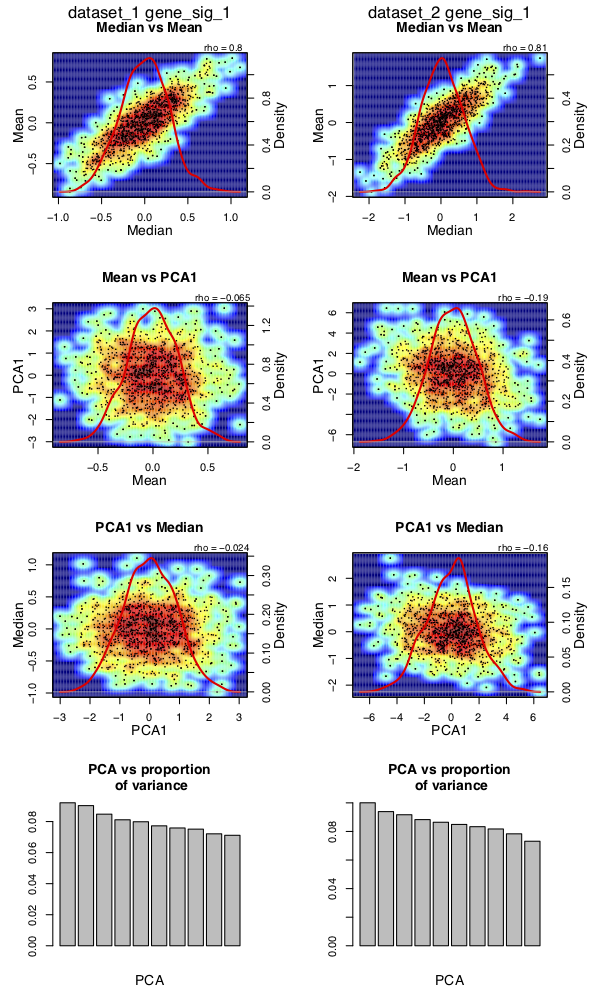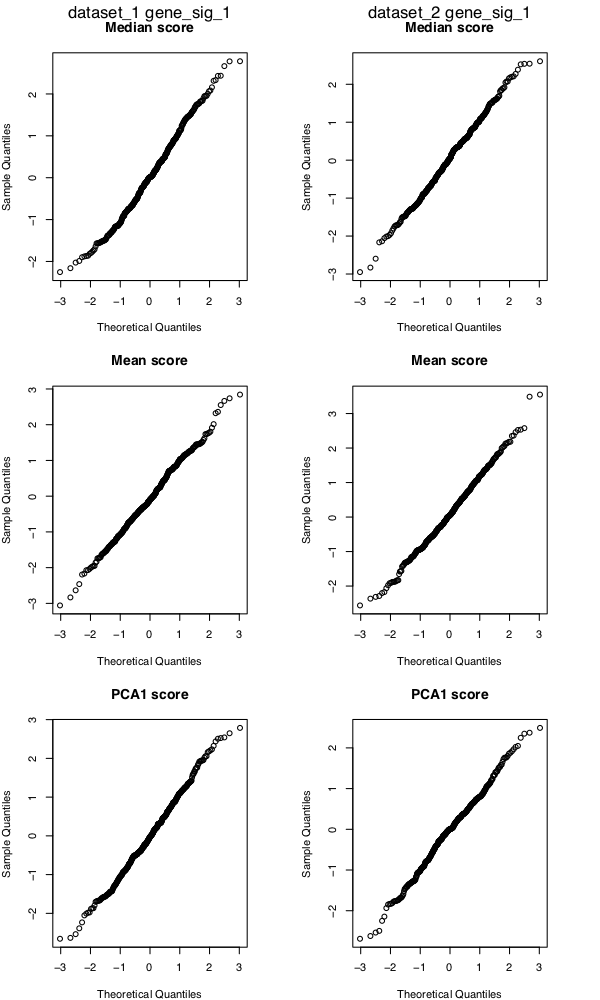# Introduction and sigQC protocol overview

Inreasing amounts of genomic data mean that gene expression signatures are becoming critically important tools, poised to make a large impact on the diagnosis, management and prognosis for a number of diseases. For the purposes of this package, we define the term gene signature to mean: ‘a set of genes whose co-ordinated mRNA expression pattern is representative of a biological pathway, process, or phenotype, or clinical outcome.’

A key issue with gene signatures of this nature is whether the expression of many genes can be summarised as a single score, or whether multiple components are represented. In this package, we have automated the testing of a number of quality control metrics designed to test whether a single score, such as the median or mean, is an appropriate summary for the genes’ expression in a new dataset. Furthermore, the tools in this package enable the visualization of properties of a set of genes in a specific dataset, such as expression profile, variability, correlation, and comparison of methods of standardisation and scoring metrics for wider scope application.s

# Package installation

To install the sigQC package, run the following code:

``install.packages('sigQC')``

# Reference

Please cite sigQC if you use it in any of your workflows:

Dhawan, Andrew, Alessandro Barberis, Wei-Chen Cheng, Enric Domingo, Catharine West, Tim Maughan, Jacob G. Scott, Adrian L. Harris, and Francesca M. Buffa. ``Guidelines for using sigQC for systematic evaluation of gene signatures.’’ Nature protocols 14, no. 5 (2019): 1377-1400.

# General overview

The sigQC package is designed to be a single function make_all_plots(), capable of customising outputs as needed for the user, based on the inputs provided.

The basic inputs are as follows:

• `gene_sigs_list`: A list of gene signatures, such that `gene_sigs_list[[sig_name]]` is a k row x 1 column character matrix for the gene set corresponding to `sig_name`
• `mRNA_expr_matrix`: A list of mRNA gene expression matrices, such that `mRNA_expr_matrix[[dataset_name]]` is a matrix with rows corresponding to genes, and columns to samples, of normalised, batch-corrected, and log-transformed (if needed), mRNA expression values
• `names_sigs`: (Optional) A vector of the names of the elements of `gene_sigs_list`
• `names_datasets`: (Optional) A vector of the names of the elements of `mRNA_expr_matrix`
• `covariates`: (Optional) A list containing a sub-list of `annotations’ and`colors’ which contains the annotation matrix for the samples in a given dataset and the associated colours, for plotting in the expression heatmap
• `thresholds`: (Optional) A list of thresholds to be considered for each data set, default is median expression of all genes of a data set. A gene is considered expressed if above the threshold, non-expressed otherwise. One threshold per dataset, in the same order as the dataset list.
• `out_dir`: (Optional) A string specifying the location/name of the directory where outputs should be saved to
• `showResults`: (Optional) A boolean specifying whether to display the output plots in R graphics windows or not
• `origin`: (Optional) A character vector with each character describing the origin of the different datasets (used only in the computation of rank product statistic among intra-signature correlation matrices, to account for batch effects)
• `doNegativeControl`: (Optional) A boolean specifying whether to do the negative and permutation control resampling for each signature/dataset combination; note that if true, it will take significantly longer to run sigQC
• `numResampling`: (Optional) A numeric value specifying the number of resampling runs to perform for the negative and permutation controls

# Sample workflow

The premise behind sigQC is to input primarily two things - the gene signatures and the datasets to test them upon. Once these inputs are prepared in the correct format, the `make_all_plots` function can be run, with settings as per user needs, generating the quality control plots needed to ascertain how each signature performs on each dataset considered.

Below, we consider the case of two random datasets and random gene signatures, and show how to customise each of the inputs.

## Step 1: Preparation of input data

Input data should consist of lists of expression matrices, and should be pre-normalised, batch-corrected, and standardised if required. Care should be taken to ensure that genes of interest are present in the dataset and not reported primarily as NA values. Gene signatures must be annotated in a manner consistent with the input data.

``````dataset_1 <- replicate(1000, rnorm(500,mean=0,sd=1))#random matrix - 1000 genes x 500 samples
row.names(dataset_1) <- as.character(1:(dim(dataset_1))) #set the gene names
colnames(dataset_1) <- paste0('d1_',as.character(1:(dim(dataset_1)))) #set the sample IDs

dataset_2 <- replicate(1000, rnorm(250,mean=0,sd=2))#random matrix - 1000 genes x 250 samples
row.names(dataset_2) <- as.character(1:(dim(dataset_2))) #set the gene names
colnames(dataset_2) <- paste0('d2_',as.character(1:(dim(dataset_2)))) #set the sample IDs

#gene sets that we are going to use for our gene signatures
gene_sig_1 <- c('1','2','3','5','8','13','21','34','55','89','144','233','377','610','987')
gene_sig_2 <- c('2','4','8','16','32','64','128','256','512')``````

Any specific expression thresholds for expression (other than global median) should be computed, as this is the default the package uses as an expression cutoff.

## Step 2: Creation of input variables

Next, we load the input datasets and gene signatures into list format.

``````mRNA_expr_matrix = list()
mRNA_expr_matrix[["dataset_1"]] = dataset_1
mRNA_expr_matrix[["dataset_2"]] = dataset_2

gene_sigs_list = list()
gene_sigs_list[['gene_sig_1']] = as.matrix(gene_sig_1)
gene_sigs_list[['gene_sig_2']] = as.matrix(gene_sig_2)``````

We then set the other non-default valued input variables as needed for our analysis:

``````showResults <- FALSE # we do not want to show the reuslts in R graphics windows
doNegativeControl <- FALSE # we do not want to compute the negative or permutation controls for time purposes``````

## Step 3: Running of sigQC package

``````library("sigQC")

make_all_plots(gene_sigs_list, mRNA_expr_matrix, showResults = showResults, doNegativeControl = doNegativeControl)``````

This will produce, in the desired output directory, a number of plots in PDF files which may be analysed as described in the subsequent steps. The package also creates an output file `log.log’ in the output directory, a text file, which summarises the run, and reports any errors that may have occurred if they are not printed to the console. This should be consulted if any issues are encountered in the running of this principal function and for troubleshooting purposes.

## Step 4: Analysis of expression

Expression of signature genes should be evaluated across samples in all datasets, and this is done by analysis of the plots sig_expr_*.pdf.

These plots describe the proportion of samples with supra-threshold expression of each signature gene (either for the median threshold, or user-specified as above), and the proportion of samples with non-NA values, identifying non-expressed signature components.Barchart of expression of signature genes above threshold

## Step 5: Analysis of variability

To check signature gene variability, load the file ‘sig_mean_vs_sd.pdf.’ These plots describe the mean and standard deviation of expression of all genes reported (in grey) versus all signature genes (in red), with corresponding dashed lines over the plots describing the 10th, 25th, 50th, 75th and 90th percentiles of both mean and standard deviation.

This facilitates the easy identification of those signature genes, which are not variable or expressed sufficiently among the samples, as well as a global evaluation of signature behaviour across samples of a dataset.Plot of mean vs. standard deviation of signature genes’ expression (red) and all other genes (grey)

## Step 6: Analysis of co-correlation of scoring metrics

Next, loading the files called ’sig_compare_metrics_*.pdf’ it is possible to analyse the co-correlation of mean, median and first principal component (PCA1) as scoring metrics across the samples for each signature across each dataset.

Also shown in the fourth row of panels of these plots is a principal components analysis (PCA) scree plot, which describes the proportion of the variance attributable to each principal component, which may reflect whether the first PCA represents a reasonable scoring summary metric for a particular gene signature.Plot of co-correlations and distributions of scoring metrics for gene signature 1 across datasets

In addition, loading the files called ’sig_compare_ES_metrics_*.pdf’, we provide analogous co-correlation plots for each of GSVA, ssGSEA, and PLAGE scores, also showing the distribution of these scoring metrics. These scores are based on the techniques implemented by the GSVA R package, which is a dependency of sigQC, and the original package and publications by Hanzelman et al., 2013, Barbie et al., 2009, and Tomfohr et al., 2005 should be consulted for details of the calculation of these metrics. As these are enrichment socre-based metrics, these are best used in concert with the quality control for signatures based on enrichment analysis results, such as those from MSigDB.

Also, loading the files called ’scoring_metrics_corr_*.pdf’, one can observe the co-correlation between all of the six scoring metrics considered (mean, median, PCA1, GSVA, ssGSEA, and PLAGE) as a heatmap of Spearman correlation coefficients. Again, this is of particular use for signatures derived based on enrichment analyses.

### Analysis of normality of scoring metrics

In the files called ’sig_qq_plots_*.pdf.’ we show QQ plots against the normal distribution, to depict how close to normally the signature scores are distributed across the datasets, for the mean, median, and first principal component.Plot of QQ plots for distributions of scoring metrics for gene signature 1 across datasets

### Analysis of modality of scoring metrics

Next, to understand the modality of the distributions of the scoring metrics across the datasets, we use the mclust package to compute the Bayes Information Criterion (BIC) for the likelihood of different Gaussian mixture models. The files called ’sig_gaussian_mixture_model_*.pdf’ provide plots of the modality on the x axis for each type of considered Gaussian mixture model, and the corresponding BIC on the y-axis.

Note that we have also provided a text output interpreting the modality for each scoring metric in each dataset/signature combination in the file ‘mixture_model_out.txt.’

Lastly, we save each of the raw ouptuts from the mclust call in the file ‘mixture_models_raw_out.rda’ in a list variable called `mixture_models`, with the following structure.

`mixture_models[[signature_name]][[dataset_name]][[metric_type]]` will contain the mclust output for the Gaussian mixture model fitting for the metric_type (one of ‘median’,‘mean’,or ‘pca1’, referring to the scoring criteria) for the dataset called ‘dataset_name’ and the signature called ‘signature_name.’